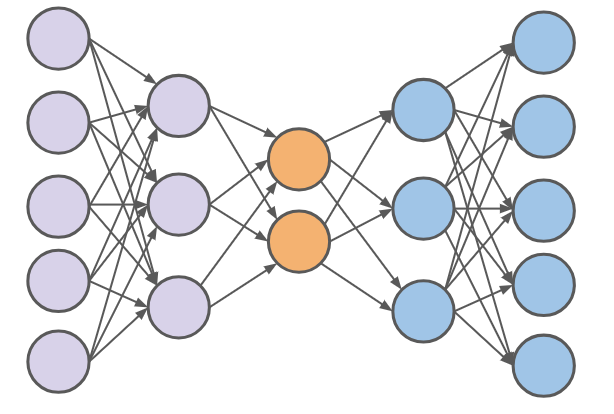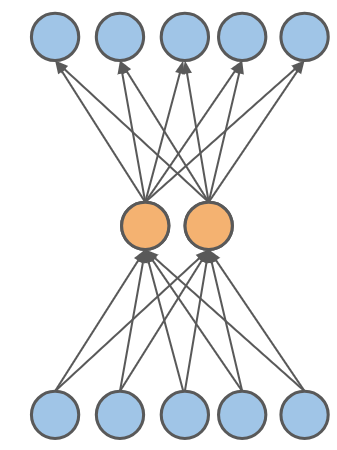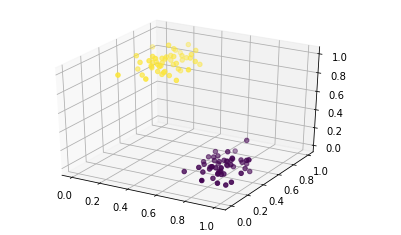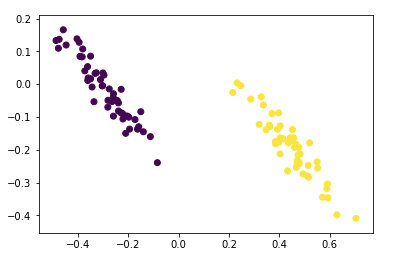AI学习笔记——Autoencoders(自编码器)

0
0
01. 云栖社区>
2. 博客>
3. 正文

AI学习笔记——Autoencoders(自编码器)

hongtao2018 2018-07-13 06:17:00 浏览363

Autoencoder 的基本概念

Autoencoder 实际上跟普通的神经网络没有什么本质的区别，分为输入层，隐藏层和输出层。唯一比较特殊的是，输入层的输入feature的数量（也就是神经元的数量）要等于输出层。同时要保证输入和输出相等。简化的Autoencoder

Autoencoder的隐藏层可以是多层也可以是单层，这里我用一个只有一层隐藏层的Autoencoder的实例来介绍Autoencoder.Autoencoder实例代码

1、导入需要用到的库
import numpy as np
import matplotlib.pyplot as plt
%matplotlib inline
2、创建一个三维的数据

from sklearn.datasets import make_blobs
data = make_blobs(n_samples=100, n_features=3,centers=2,random_state=101)3. 搭建神经网络

import tensorflow as tf
from tensorflow.contrib.layers import fully_connected

num_inputs = 3  # 3 dimensional input
num_hidden = 2  # 2 dimensional representation
num_outputs = num_inputs # Must be true for an autoencoder!

learning_rate = 0.01

Placeholder,Layers,Loss Function 和 Optimizer

#Placeholder
X = tf.placeholder(tf.float32, shape=[None, num_inputs])
#Layers
hidden = fully_connected(X, num_hidden, activation_fn=None)
outputs = fully_connected(hidden, num_outputs, activation_fn=None)
#Loss Function
loss = tf.reduce_mean(tf.square(outputs - X))  # MSE
#Optimizer
train  = optimizer.minimize( loss)
#Init
init = tf.global_variables_initializer()

4. 训练神经网络

num_steps = 1000

with tf.Session() as sess:
sess.run(init)

for iteration in range(num_steps):
sess.run(train,feed_dict={X: scaled_data})

# Now ask for the hidden layer output (the 2 dimensional output)
output_2d = hidden.eval(feed_dict={X: scaled_data})4.总结

————

AI学习笔记——循环神经网络（RNN）的基本概念
AI学习笔记——神经网络和深度学习
AI学习笔记——卷积神经网络1（CNN）
————

hongtao2018
+ 关注